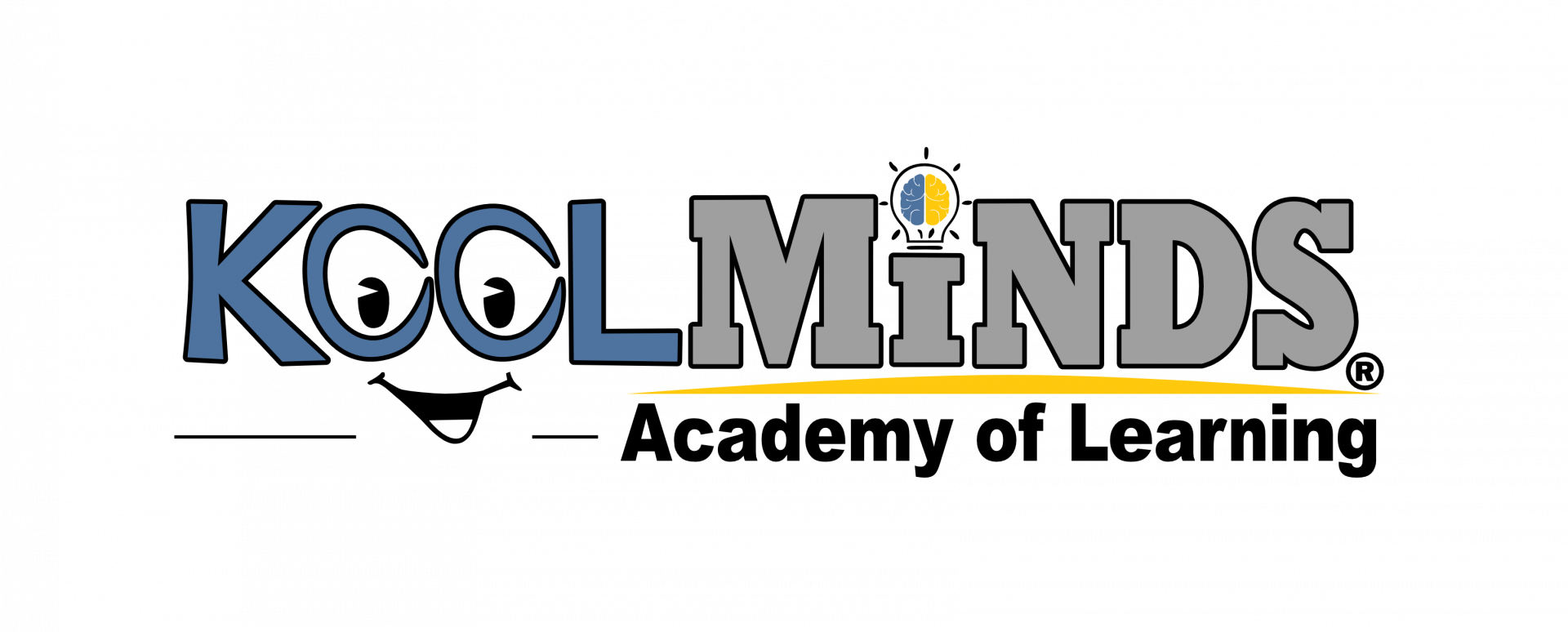## Call or Text 866.566.5637

KoolMinds Multisensory math is a complete and comprehensive K-12 math curriculum. Each level concentrates on a specific set of skills, while continuously reviewing and integrating topics and concepts presented in previous levels. These tools are designed to appeal to all types of learners in a variety of learning environments. We start with introductory math skills all the way through to Calculus.

• Level 1: 30 Lessons; Numbers
• Level 2: 30 Lessons; Addition & Subtraction
• Level 3: 30 Lessons; Addition & Subtraction Multiple Digits
• Level 4: 30 Lessons; Multiplication
• Level 5: 30 Lessons; Division
• Level 6: 30 Lessons; Fractions
• Level 7: 30 Lessons; Decimals & Percent's
• Level 8: 30 Lessons; Pre-Algebra: Negative Numbers
• Level 9: 35 Lessons: Algebra: Graphics, Equations, Exponents, Polynomials
• Level 10: 30 Lessons; Geometry: Points, Lines, Angles, Circles, and More…
• Level 11: 31 Lessons; Algebra 2: Factoring Polynomials, Quadratic, etc.…
• Level 12: 30 Lessons; Pre-Calculus: Trigonometry, Identities, Polar Equation, Logarithms, Sequences, Limits, and More…
• Level 13: 30 Lessons; Calculus: Derivatives, Integrals, Calculus, etc.….

Integrated Manipulatives – Conceptual Understanding – Simple Design – Skill-Based Design